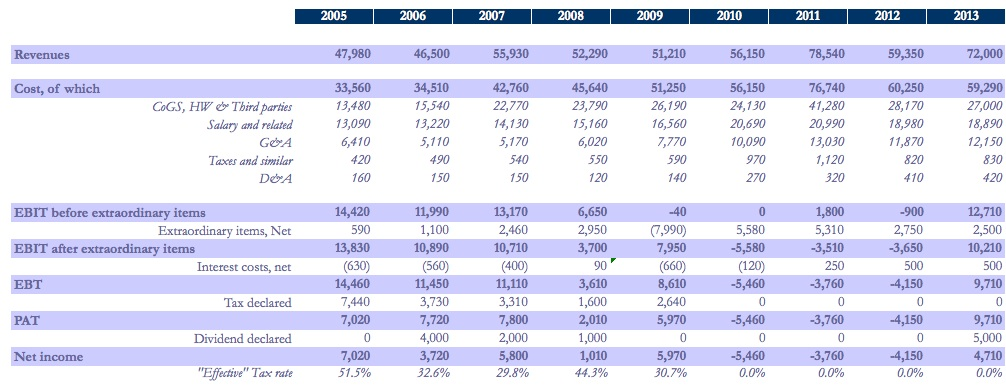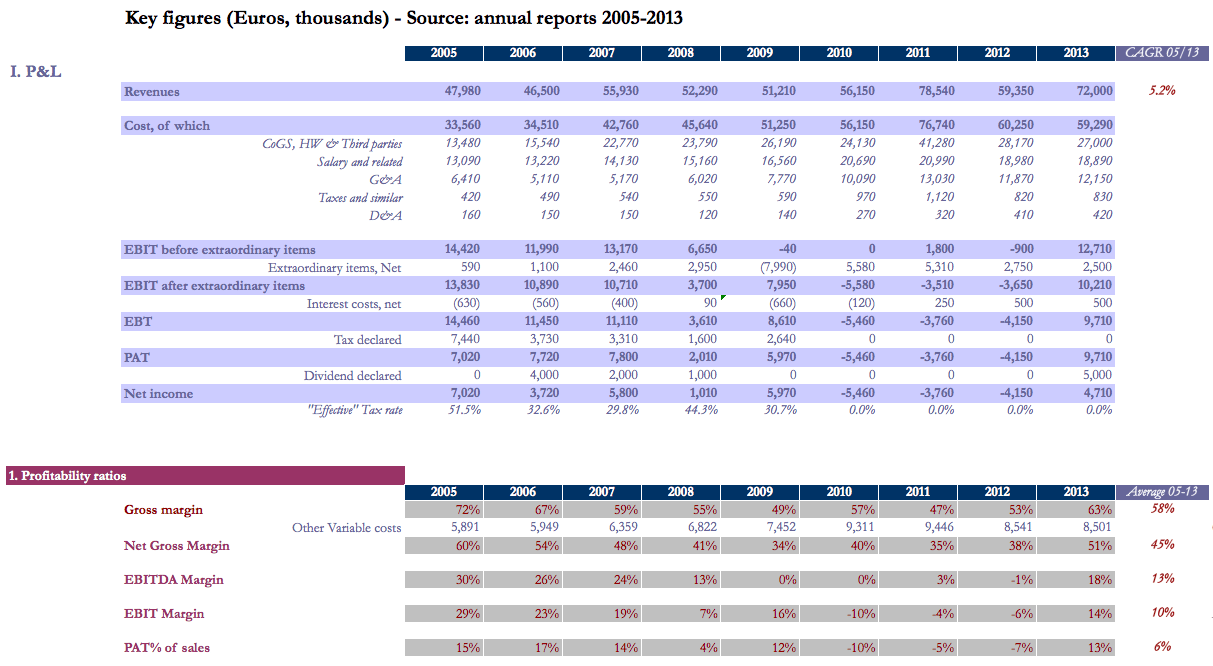There are 4 main categories of financial ratios and KPIs used by financial practitioners, each addressing a specific question:

Question 1: “Is the business profitable?” -> Profitability ratios, calculated from the P&L (e.g. Gross margin, EBITDA margin, EBIT margin)

Question 2: “Is the business liquid in the short term?” -> Liquitidity ratios, calculated from the Balance Sheet (e.g. current ratio, liquid ratio, cash ratio)

Question 3: “Is the business financially stable in the long term?” –> Stability ratios, calculated from the Balance Sheet (e.g. debt-to-equity ratio, gearing, debt cover ratio)

Question 4: “Is profitability high enough compared to what we have invested?” –> Capital Efficiency ratios (e.g. ROE taking an ‘equity’ perspective; ROIC taking an ‘entity’ point of view).

In additional, practitioners often undertake a Cost Structure Analysis, as well as a Working Capital Analysis (e.g. receivables days, inventory days, payable days).

This analysis is not hard when you understand the meaning of these ratios, and how to calculate them. To help you get started, Investaura Management Consultants is pleased to provide you with this Financial Ratio Analysis template.